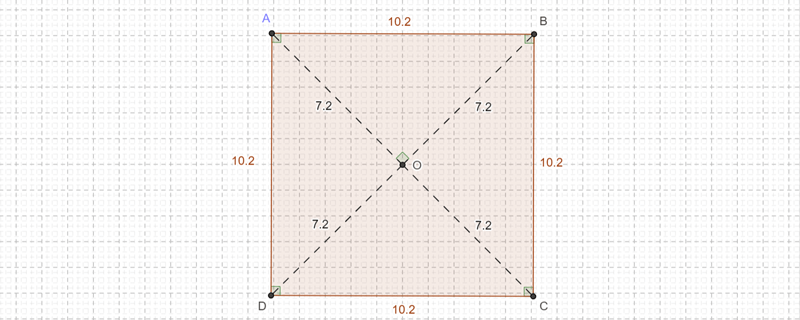# The geometer's warehouse

Secondary KLA:
Mathematics
Educational levels:
Year 7, Year 8, Year 9, Year 10This web-based, multimedia resource focuses on the geometry of the Stage 4 and Stage 5 Mathematics syllabus. It comprises 70 dynamic html worksheets, each exploring a different outcome in Stage 4 and Stage 5 geometry.

## NSW syllabus outcomes

(MA3-16MG) measures and constructs angles, and applies angle relationships to find unknown angles

(MA3-15MG) manipulates, classifies and draws two-dimensional shapes, including equilateral, isosceles and scalene triangles, and describes their properties

(MA4-17MG) classifies, describes and uses the properties of triangles and quadrilaterals, and determines congruent triangles to find unknown side lengths and angles

(MA4-18MG) identifies and uses angle relationships, including those related to transversals on sets of parallel lines

(MA5.2-14MG) calculates the angle sum of any polygon and uses minimum conditions to prove triangles are congruent or similar

(MA5.3-16MG) proves triangles are similar, and uses formal geometric reasoning to establish properties of triangles and quadrilaterals

(MA5.3-17MG) applies deductive reasoning to prove circle theorems and to solve related problems

## Australian curriculum content descriptions

(ACMMG141) Investigate, with and without digital technologies, angles on a straight line, angles at a point and vertically opposite angles. Use results to find unknown angles

(ACMMG163) Identify corresponding, alternate and co-interior angles when two straight lines are crossed by a transversal

(ACMMG164) Investigate conditions for two lines to be parallel and solve simple numerical problems using reasoning

(ACMMG166) Demonstrate that the angle sum of a triangle is 180° and use this to find the angle sum of a quadrilateral

(ACMMG202) Establish properties of quadrilaterals using congruent triangles and angle properties, and solve related numerical problems using reasoning

(ACMMG244) Apply logical reasoning, including the use of congruence and similarity, to proofs and numerical exercises involving plane shapes

(ACMMG272) Prove and apply angle and chord properties of circles

Resource type:
Interactive Resource
Audience:
learner
ScOT topics:
Geometry, Angles, Theorems
File type:
text/html
Language/s:
en-AU
Author:
State of NSW, Department of Education
Publisher:
State of NSW, Department of Education
Date created:
Thursday, 2 August 2018

Resource ID: cb02edfa-eb1d-43e7-b913-08e55cdb3435